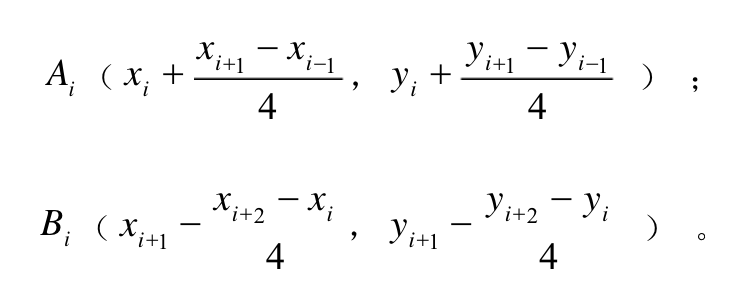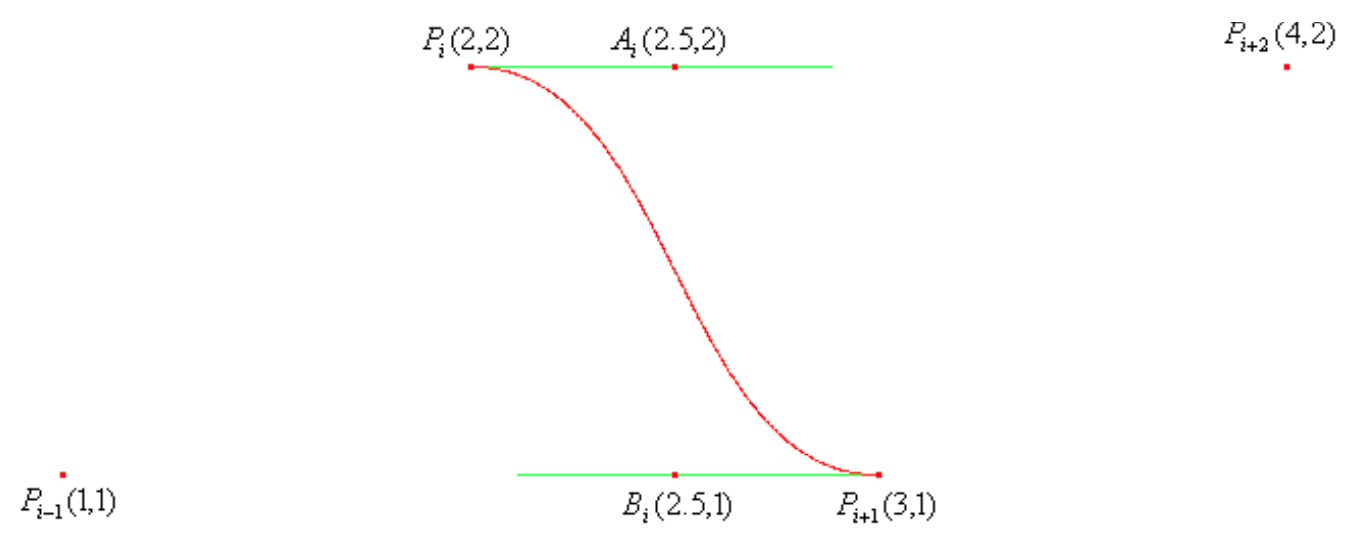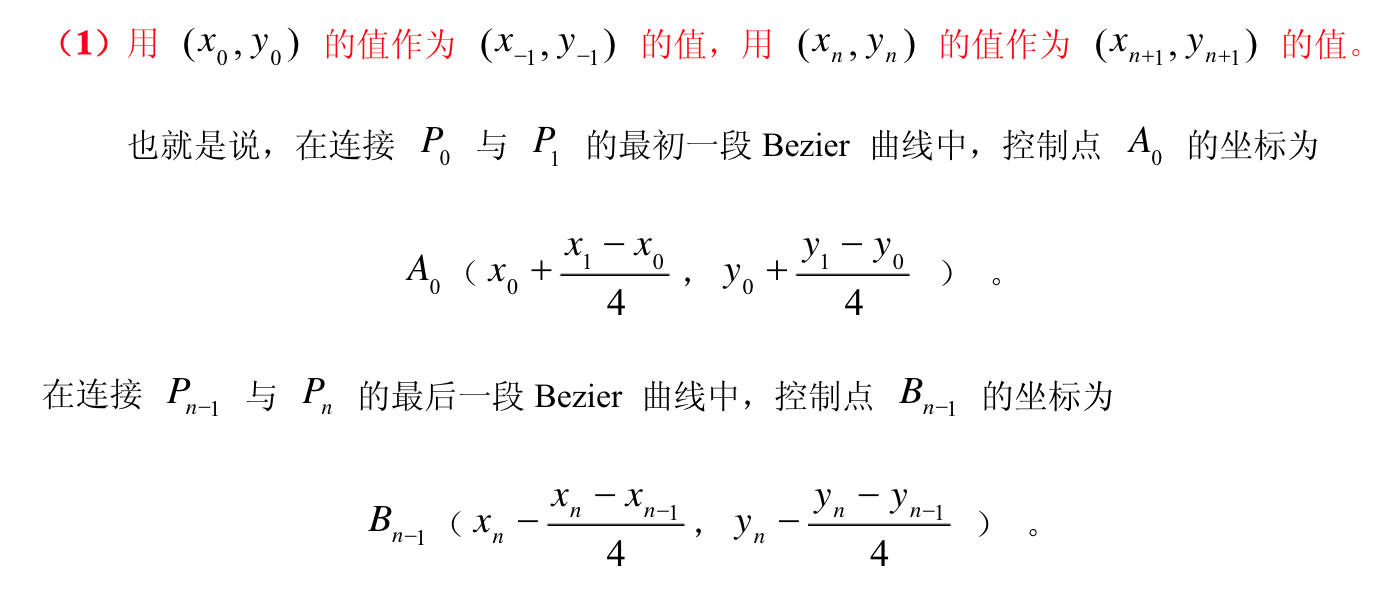# 基于canvas使用贝塞尔曲线平滑拟合折线段

## 实现过程

• 绘制折线图
• 贝塞尔曲线平滑拟合

#### 模拟数据

``````
var data = [Math.random() * 300];
for (var i = 1; i < 50; i++) { //按照echarts
data.push(Math.round((Math.random() - 0.5) * 20 + data[i - 1]));
}
option = {
canvas:{
id: 'canvas'
},
series: {
name: '模拟数据',
itemStyle: {
color: 'rgb(255, 70, 131)'
},
areaStyle: {
color: 'rgb(255, 158, 68)'
},
data: data
}
};
``````

#### 绘制折线图

``````
function LinearGradient(option) {
this.canvas = document.getElementById(option.canvas.id)
this.ctx = this.canvas.getContext('2d')
this.width = this.canvas.width
this.height = this.canvas.height
this.tooltip = option.tooltip
this.title = option.text
this.series = option.series //存放模拟数据
}
``````

``````
LinearGradient.prototype.draw1 = function() { //折线参考线
...
//要考虑到canvas中的原点是左上角，
//所以下面要做一些换算，
//diff为x,y轴被数据最大值和最小值的取值范围所平分的等份。
this.series.data.forEach(function(item, index) {
var x = diffX * index,
y = Math.floor(self.height - diffY * (item - dataMin))
self.ctx.lineTo(x, y) //绘制各个数据点
})
...
}
``````

### 贝塞尔曲线平滑拟合所以在将折线换成平滑曲线的时候，将边界值以及其他控制点计算好之后代入到贝塞尔函数中就完成了：

``````
//核心实现
this.series.data.forEach(function(item, index) { //找到前一个点到下一个点中间的控制点
var scale = 0.1 //分别对于ab控制点的一个正数，可以分别自行调整
var last1X = diffX * (index - 1),
last1Y = Math.floor(self.height - diffY * (self.series.data[index - 1] - dataMin)),
//前一个点坐标
last2X = diffX * (index - 2),
last2Y = Math.floor(self.height - diffY * (self.series.data[index - 2] - dataMin)),
//前两个点坐标
nowX = diffX * (index),
nowY = Math.floor(self.height - diffY * (self.series.data[index] - dataMin)),
//当期点坐标
nextX = diffX * (index + 1),
nextY = Math.floor(self.height - diffY * (self.series.data[index + 1] - dataMin)),
//下一个点坐标
cAx = last1X + (nowX - last2X) * scale,
cAy = last1Y + (nowY - last2Y) * scale,
cBx = nowX - (nextX - last1X) * scale,
cBy = nowY - (nextY - last1Y) * scale
if(index === 0) {
self.ctx.lineTo(nowX, nowY)
return
} else if(index ===1) {
cAx = last1X + (nowX - 0) * scale
cAy = last1Y + (nowY - self.height) * scale
} else if(index === self.series.data.length - 1) {
cBx = nowX - (nowX - last1X) * scale
cBy = nowY - (nowY - last1Y) * scale
}
self.ctx.bezierCurveTo(cAx, cAy, cBx, cBy, nowX, nowY);
//绘制出上一个点到当前点的贝塞尔曲线
})
``````

## 最后

• 点赞
• 写回答
• 关注问题
• 收藏
• 复制链接分享
• 邀请回答

#### 8条回答

• 打扰了，看起来像Android的kotlin

点赞 评论 复制链接分享
• 受教了受教了

点赞 评论 复制链接分享
• 持续关注中....

点赞 评论 复制链接分享
• hi，请问，你的github的blog是用什么写的啊？markdown么？

点赞 评论 复制链接分享
• markdown

点赞 评论 复制链接分享
• diff是啥意思 没看明白

点赞 评论 复制链接分享
1. PathEffect mEffect = new CornerPathEffect(20);//平滑过渡的角度 2.mPaint.setPathEffect(mEffect); 3.canvas.drawPath(path, mPaint); 直接用path实现，不用计算
点赞 评论 复制链接分享
• 大佬我百度了一下这个写法好像是安卓的0.0 我不太会233 我是用的html5的canvas..

点赞 评论 复制链接分享